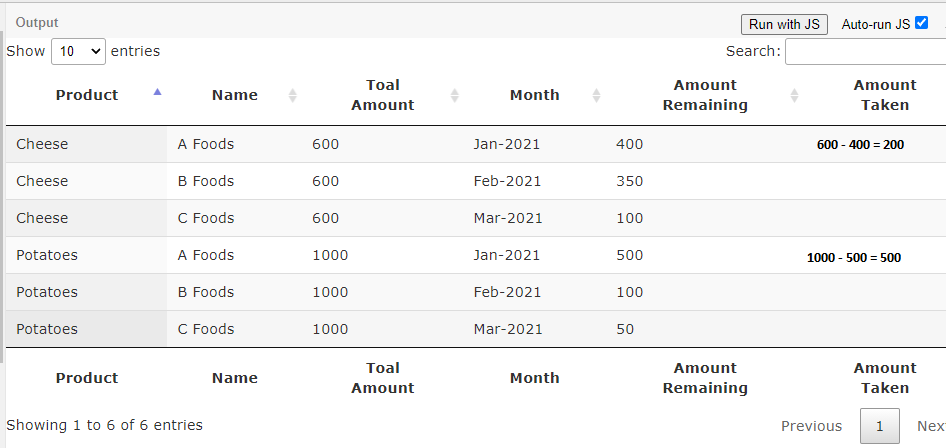# Computed values to calculate value of a column

I have a very simple tableTotal amount corresponds to the total amount in units which is agreed, each month if units are taken/consumed the column (amount remaining ) is updated with the amount of units remaining. Based on these two columns , I need to calculate the amount taken which will be Total Amount - Amount Remaining = Amount Taken

For the first row it is easy to calculate, Total Amount(600) - Amount Remaining(400) = Amount Taken(200) . However, for the next row the value for Total amount is to be taken from row above for example : Total Amount(400) - Amount Remaining(350) = Amount Taken(50) .
How can that be achieved? of is there a simple way of doing it ?

Thank you